• c语言实现后缀表达式
2021-05-20 10:00:25

使用栈来进行后缀表达式计算，流程：从前向后读取后缀表达式的项目，遇到数值压入栈中，遇到运算符pop出栈顶的两项做运算，运算结果再放入栈中直到=

例子：求后缀表达式4x*2x*a-c*+=？

1.把4和x压入栈中接下来遇到了运算符*把4和x取出做*运算得到4x放入栈中，此时栈中有4x

2.接下来2和x放入栈中遇到了运算符*把2和x取出做*运算得到2x放入栈中，此时栈中有4x，2x

3.接下来遇到了a把a入栈，栈里项目为4x，2x，a，遇到预算符-，取出2x和a做-运算得到2x-a再放入栈，此时栈里是4x，2x-a

4.接下来把c压入栈，栈里项目为4x，2x-a，c，遇到预算符*，取出2x-a和c，*运算得到(2x-a)c，再入栈，此时栈里是4x，(2x-a)c

5.最后遇到+运算符，把栈中4x，(2x-a)c做+运算得到最终结果4x+(2x-a)c

以上就是运用栈求后缀表达式的流畅，下面我们来用代码实现它：

(代码来源：http://blog..net/woshinannan741/article/details/50087665)

#include

#include

#include

#include

#include

#include

#define MAXN 1000

using namespace std;

stack s;  //定义了一个栈

char *tranfExp(char* exp)

{

char tempStr;//保存后缀表达式的字符串

int i=0,j=0;

while(exp[i] !='\0')

{

if(exp[i]>='0' &&exp[i]<='9')  //如果是数字字符串就保存到后缀表达式字符串中

{

tempStr[j++] = exp[i];

}

else if(exp[i] == '(' )  //如果是左括号及入栈

{

s.push(exp[i]);

}

else if(exp[i] == ')' )  //如果是右括号就把接近栈顶的左括号上面所有的运算符出栈存进字符串中  左括号出栈

{

while(s.empty() == false)

{

if(s.top() == '(' )

{

s.pop();

break;

}

else

{

tempStr[j++] = s.top();

s.pop();

}

}

}

else if(exp[i] == '+' || exp[i] == '-')   //如果的事+-|操作符就把比他优先级高或者等于的所有运算符出栈进入字符串

{

while(s.empty() == false)

{

char ch = s.top();

if(ch == '+'||ch == '-'||ch == '/'||ch == '*')

{

tempStr[j++] = s.top();

s.pop();

}

else

break;

}

s.push(exp[i]);

}

else if(exp[i] == '*' || exp[i] == '/')  //类似于扫描到+- 只是如果栈中有=-运算符就不用出栈  因为运算符优先级比较小

{

while(s.empty() == false)

{

char ch = s.top();

if(ch == '/' || ch=='*')

{

tempStr[j++] = s.top();

s.pop();

}

else

break;

}

s.push(exp[i]);

}

i++;

}

while(s.empty() == false)   //把栈中剩余的所有运算符出栈

{

tempStr[j++] = s.top();

s.pop();

}

tempStr[j] = 0;   //最后一个赋值为0  也就是字符串结束的标志

return tempStr;   //返回已经得到的后缀表达式

}

int calcExp(char* exp)// 计算后缀表达式

{

puts(exp);   //展示已经得到的后缀

while( !s.empty() )

s.pop();

int i=0;

while(exp[i] != '\0')

{

if(exp[i]>='0' && exp[i]<='9')

{

s.push(exp[i]-'0');

}

else if(exp[i] == '-')

{

int m = s.top();

s.pop();

int n = s.top();

s.pop();

s.push(n-m);

}

else if(exp[i] == '+')

{

int m = s.top();

s.pop();

int n = s.top();

s.pop();

s.push(n+m);

}

else if(exp[i] == '/')

{

int m = s.top();

s.pop();

int n = s.top();

s.pop();

s.push(n/m);

}

else if(exp[i] == '*')

{

int m = s.top();

s.pop();

int n = s.top();

s.pop();

s.push(n*m);

}

i++;

}

/* while(s.empty() == false)

{

printf("%d",s.top());

s.pop();

}*/

printf("\n\n\n");

return s.top();

}

int main()

{

char str;

char* tranStr;

tranStr = (char *)malloc(100*sizeof(char));

// scanf("%s",str);

str='2*(3+2)-5*2';

tranStr =  tranfExp(str);//中缀表达式转换为后缀表达式函数

//puts(tranStr); //输出转换后的后缀表达式

printf("%d",calcExp(tranStr));

return 0;

}

更多相关内容
• 主要为大家详细介绍了C语言实现中缀表达式转换为后缀表达式，文中示例代码介绍的非常详细，具有一定的参考价值，感兴趣的小伙伴们可以参考一下
• C语言 实现后缀表达式求值（理解不同方式）

# 写在前面

在数据结构中，栈是非常重要的储存结构，比如栈应用于求表达式，将输入的等式进行转换，如前缀表达式、后缀表达式等然后进行求值并返回结果。

但是本文并为使用栈实现后缀表达式求值，而是通过一般函数方法，需要先计算一下，将原式转换成后缀表达式后再输入求值哦，试试看。

# 代码实现

#include <stdio.h>
#include <stdlib.h>

void evalPf(char*);
double cal(double, char, double);

int main(){
char input;
printf("请输入后缀式：\n\n");
printf("例如：\n算式 (1+2)*(3+4) 的后缀式为：12+34+*  \n\n");
scanf("%s", input);
evalPf(input);
return 0;
}

void evalPf(char* postfix){
double stack = {0.0};
char temp;
char token;
int top = 0, i = 0;
temp = '\0';
while(1){
token = postfix[i];
switch(token){
case '\0':
printf("原式 = %f\n", stack[top]);
return;
case '+':
case '-':
case '*':
case '/':
stack[top-1] =cal(stack[top], token, stack[top-1]);
top--;
break;
default:
if(top < sizeof(stack) / sizeof(float)){
temp = postfix[i];
top++;
stack[top] = atof(temp);
}
break;
}
i++;
}
}

double cal(double p1, char op, double p2){
switch(op){
case '+':
return p1 + p2;
case '-':
return p1 - p2;
case '*':
return p1 * p2;
case '/':
return p1 / p2;
}
}

# 运行结果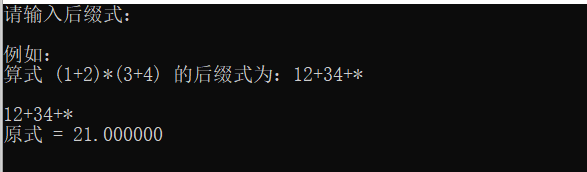展开全文数据结构 算法
• c语言实现中缀表达式转后缀表达式并求得计算结果，用顺序栈结构。 当输入者输入错误信息的时候需要报错，并说明错误的种类。
• C语言用栈实现 中缀表达式转后缀表达式 数据结构

# 全部代码如下：懂意思就好

#include <stdio.h>
#include <stdlib.h>
#include<stdbool.h>
#define MaxSize 40
typedef struct
{
char data[MaxSize];
int top;

} Stack,*Stacks;

Stack * init_Stack(Stacks s)//初始化栈
{
s=(Stack*)malloc(sizeof(Stack));
s->top=-1;
printf("初始化成功!\n");
return s;
}
Stack * push(Stacks s,char x)//入栈
{

if(s->top==MaxSize-1)
{
printf("栈满!\n");
}
else
{
s->top=s->top+1;
s->data[s->top]=x;

}
return s;
}
char pop(Stacks s)//出栈
{
char x='0';

if(s->top==-1)
{
printf("使用pop导致栈空!\n");
}
else
{
x=s->data[s->top];
s->top=s->top-1;
}
return x;
}
char get_top(Stacks s)//取栈顶元素
{
char x='0';

if(s->top==-1)
{
printf("栈空!\n");
}
else
{
x=s->data[s->top];
}
return x;
}

int judge(char s)//比较当前扫描到的是数字还是运算符还是括号
{

if(s>='1' && s<='9' ) //49~57
{
return 1;//表示是数字
}
else if(s=='(')
{
return 2;//表示左括号
}
else if(s==')')
{
return 3;//表示右括号
}
else
{
return 4;//表示运算符
}

}
bool compare(char a,char b)//运算符之间优先级比较
{
if((a=='+' || a=='-') &&( b=='+' || b=='-'))//同级和比栈顶低一级，都执行相同的操作:出栈
{
return true;//表示同级
}
else if((a=='+' || a=='-') && (b=='*' || b=='/'))
{
return true;//表示低一级
}
else if((a=='*' || a=='/') && (b=='*' || b=='/'))
{
return true;//表示同级
}
else if((a=='*' || a=='/') && (b=='+' || b=='-'))
{
return false;//表示比栈顶高一级，则入栈

}

}
void display(Stacks s)//显示栈内元素
{
int i=1;
int temp=s->top;//保存指向栈顶的位置，方便复原
while(s->top!=-1)
{
printf("data%d=%c\n",i++,s->data[s->top--]);
}

s->top=temp;

}
void middle_to_postfix(char str1[],int length) //中缀转后缀
{
Stacks s;
s=init_Stack(s);
char str2[length];//用来保存后缀表达式
int j=0;//当作str2的下标
for(int i=0; i<length; i++)
{
if(judge(str1[i]) == 1) //如果是数字，则直接放进str2
{
str2[j++]=str1[i];
}
else//扫描到的不是数字
{
if(s->top==-1 || judge(str1[i])==2 ) //如果栈空，表明之前没有符号，直接将符号入栈;左括号也直接入栈
{
s=push(s,str1[i]);
}
else if(judge(str1[i])==3)   //当前扫描到右括号，则出栈，遇到左括号为止
{
while(get_top(s)!='(')
{
str2[j++]=pop(s);
}
pop(s);//最后再把左括号出栈
}
else if(judge(str1[i])==4)  //栈不为空，且当前扫描到的是运算符，比较当前运算符与栈顶运算符的运算优先级
{

if(s->data[s->top]=='(') //栈顶是左括号，直接入栈
{
push(s,str1[i]);
}
else if(compare(str1[i],get_top(s)) && s->top!=-1) //是同级，或比栈顶低一级，则出栈，
{
str2[j++]=pop(s);
push(s,str1[i]);//再把当前同级的符号入栈
}
else  //比栈顶元素，高一级则入栈
{
s=push(s,str1[i]);
}

}
}

}
while(s->top!=-1)//将栈内剩余的全部输出
{
str2[j++]=s->data[s->top--];
}
for(int i=0; i<j; i++)//显示最后的后缀表达式
{
printf("%c ",str2[i]);
}

}
int main()
{

char a= {0};
gets(a);
int length=0;
while(a[length]!='\0')
{
printf("已输入:%c\n",a[length++]);

}
middle_to_postfix(a,length);

return 0;
}


展开全文c++ 算法 数据结构
• 利用C语言实现中缀表达式转化为后缀表达式！！利用栈。
• 本文实例为大家分享了C语言实现后缀表达式（逆波兰表达式）的求解代码，供大家参考，具体内容如下 逆波兰表达式： 逆波兰表达式又叫后缀表达式。它是由相应的语法树的后序遍历的结果得到的。 例：5 – 8*(6 + 7) +...
• 中缀表达式到后缀表达式的转换是堆栈应用的典型例子。通过只允许操作‘+’，‘-’，‘*’，‘/’，并坚持优先级法则，即可实现该操作。 1. 规则 假设读入的中缀表达式是正确的。 当读到操作数时，立即输出。 操作符...

中缀表达式到后缀表达式的转换是堆栈应用的典型例子。通过只允许操作‘+’，‘-’，‘*’，‘/’，并坚持优先级法则，即可实现该操作。

## 1. 规则

假设读入的中缀表达式是正确的。
当读到操作数时，立即输出。
操作符不立即输出，而是放入栈中。定义‘（’的优先级最高，其余符号同四则运算的法则。读入一个操作符时，弹出栈顶元素直至发现优先级更低的元素为止。除非是在处理一个‘）’，否则绝不从栈中弹出‘（’。遇到‘）’，将栈顶元素弹出并输出直到遇到相应的‘（’，但该‘（’只弹出，不输出。
读入输入的末尾时，若栈非空，依此弹出并输出所有栈元素。

## 2. 实例

以中缀表达式1+（2 -3 ）*4+5 / 6 为例：
首先读入1，并输出。接着读入‘+’，此时栈为空，将‘+’压入栈中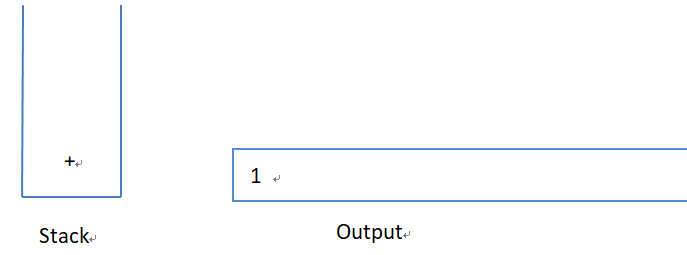这时读入‘（’，由于栈顶元素‘+’比‘（’的优先级低，故没有输出，‘（’进栈。接着，2被读入并输出。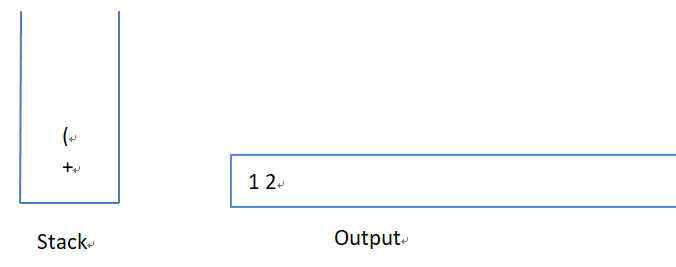接着‘-’读入，除非在处理‘）’，否则‘（’不会从栈中弹出。因此将‘-’压入栈中。下一个是3，被读入并输出。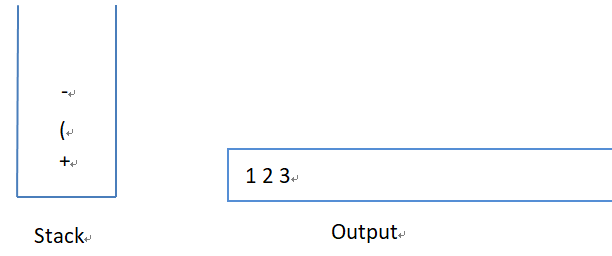此时读入‘）’，因此将栈元素直到‘（’弹出，输出一个‘-’。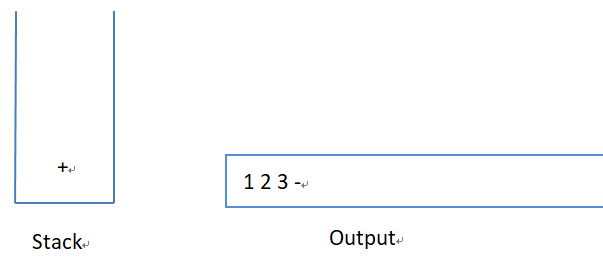接着读入‘’，栈顶元素‘+’优先级较低，故将乘号压入栈中。同时4被读入并输出。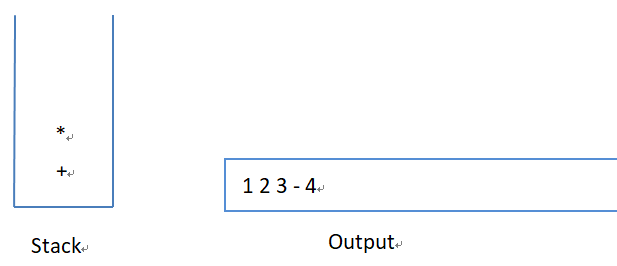继续进行，‘+’被读入，此时需要把‘
’从栈弹出并输出；现在栈顶元素为‘+’，该算符不比刚刚遇到的‘+’优先级低而是具有相同的优先级，因而被弹出并放入输出中。然后，将谷歌遇到的‘+’压入栈中。同时5被读入并输出。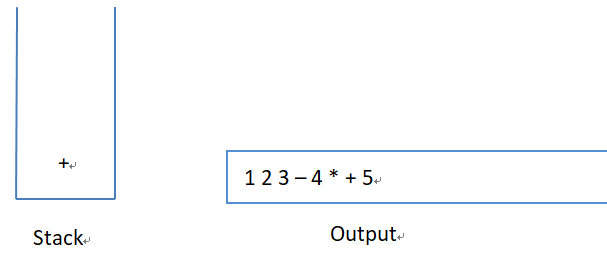现在，读入‘/’，该算符优先级高于栈顶元素，因而被压入栈中。再往后读入6，并将其输出。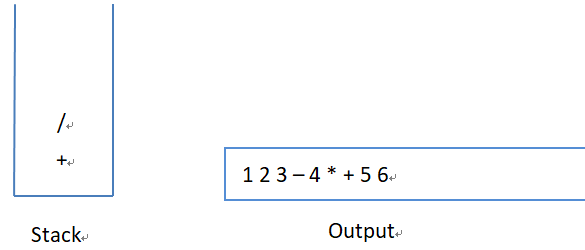至此，输入为空，因而将栈元素全部弹出并输出，直至栈为空。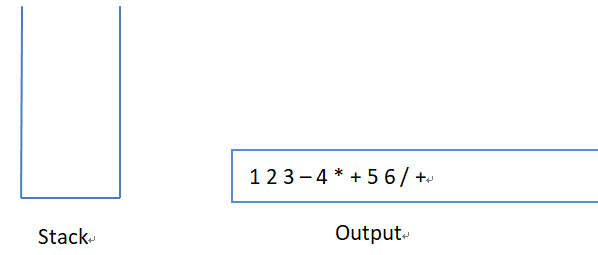## 3. 代码

#include<stdio.h>
#include<stdlib.h>
#include<string.h>

#define EmptyTOS -1        //栈空时指针指向
#define MinStackSize 5     //栈的最小容量
#define MaxArraySize  50   //待读取数组初始最大容量

typedef struct StackRecord* Stack;

struct StackRecord
{
int capacity;          //栈容量
int TopOfStack;        //栈顶
char* array;
};

Stack CreateStack(int size)        //创建空栈
{
Stack s;
if (size < MinStackSize)
{
printf("The size is too small!\n");
return;
}
s = (Stack)malloc(sizeof(struct StackRecord));
s->capacity = size;
s->TopOfStack = EmptyTOS;
s->array = (char*)malloc(sizeof(char) * size);

return s;
}

int IsEmpty(Stack s)
{
return s->TopOfStack == EmptyTOS;
}

int IsFull(Stack s)
{
return s->TopOfStack == s->capacity - 1;
}

void Push(Stack s, char c)        //压栈
{
if (IsFull(s))
{
printf("Error: the Stack is full!");
return;
}
else
{
s->array[++(s->TopOfStack)] = c;
}
}

char Top(Stack s)      //返回栈顶元素，不弹出
{
if (IsEmpty(s))
{
printf("Error: the Stack is empty!");
return;
}
else
return s->array[s->TopOfStack];
}

char Pop(Stack s)        //弹出栈顶元素
{
if (IsEmpty(s))
{
printf("Error: the Stack is empty!");
return;
}
else
return s->array[s->TopOfStack--];
}

int IsHigherThan(char x, char y)       //优先级定义，'('具有最高优先级，其余同四则运算
{
if ((x == '(') && ((y == '+') || (y == '-') || (y == '*') || (y == '/')))
return 1;
else if (((x == '*') || (x == '/')) && ((y == '+') || (y == '-')))
return 1;
else
return 0;
}

void InfixToPostfix(char* a)       //中缀转后缀
{
Stack s = CreateStack(50);
int i = 0;

while (a[i]!='\0')
{
if ((a[i] >= '0' && a[i] <= '9') || (a[i] >= 'a' && a[i] <= 'z') || (a[i] >= 'A' && a[i] <= 'Z'))
printf("%c ", a[i]);      //数字直接输出
else if (a[i] == ')')
{
while (Top(s) != '(')
printf("%c ", Pop(s));       //遇到右括号，弹出并输出栈顶元素直到遇到左括号（左括号不输出）
Pop(s);
}
else
{
if (IsEmpty(s))   //栈空则直接推入栈中
Push(s, a[i]);
else if (IsHigherThan(a[i], Top(s)))     //优先级高则推入栈中
Push(s, a[i]);
else
{
while (!IsEmpty(s) && (!IsHigherThan(a[i], Top(s))))   //弹出栈顶元素直至遇到优先级更低的元素
{
if (Top(s) != '(')    //只有遇到右括号才能将左括号弹出
printf("%c ", Pop(s));
else
break;
}
Push(s, a[i]);
}
}
i++;
}

while (!IsEmpty(s))    //输入读取完毕栈非空，则依此弹出所有栈元素
{
printf("%c ", Pop(s));
}
}

int main()
{
char str[MaxArraySize];
printf("Enter the infix:\n");
gets(str);
printf("The postfix:\n");
InfixToPostfix(str);

return 0;
}


## 4.测试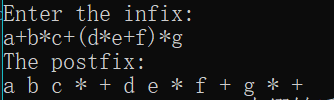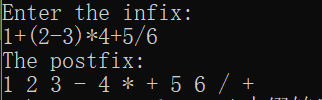展开全文堆栈 数据结构 算法
• 后缀表达式什么是后缀表达式后缀表达式计算功能快捷键合理的创建标题，有助于目录的生成如何改变文本的样式插入链接与图片如何插入一段漂亮的代码片生成一个适合你的列表创建一个表格设定内容居中、居左、居右...
• 后缀表达式计算请使用已定义好的栈完成后缀表达式计算： (1)如果是操作数，直接入栈 (2)如果是操作符op，连续出栈两次，得到操作数x 和 y,计算 x op y，并将结果入栈。后缀表达式示例如下： 9 3 1 - 3 * + 10 2 /...
• } //表达式运算符优先级低则执行出栈操作，弹出的栈顶元素成为后缀表达式的一部分 //当遇到“）”，则执行出栈操作直到“（”，但括号不计入后缀表达式中 while (!IsEmpty(Stack) && JudgeLevel(0, a[i]) (1, Stack...stack 数据结构
• 本文实例为大家分享了C++实现中缀表达式转后缀表达式的具体代码，供大家参考，具体内容如下 题目：现有中缀表达式如：1+(2-3)*4+10/5 请用栈的特性编写一个程序，使得程序输出后缀表达式 分析如下： STEP1： 1+(2-3)...
• C语言后缀表达式计算一、设计思想计算算数表达式并求值，采取的共有两种方法：先将算数表达式转化为后缀表达式，然后对后缀表达式进行计算。对算数表达式进行直接的计算。第一种算法这种解决方案又分为两步：1.将...
• C语言计算后缀表达式，从控制台输入，以空格为分割输入，支持多位数和负数运算，支持加、减、乘、除、阶乘操作，支持表达式错误检测异常。 input： 3 1 -2 * 1 10 - 6 + 3 1 - ！ Output： -2 -3 2 #include<...开发语言
• } /* 函数名：inToPost 函数功能：将中缀表达式转换为后缀表达式输出 函数参数：中缀表达式，放在字符数组中 返回值：无 */ int inToPost(char *expression) { //在此处填写代码，完成中缀表达式转换为后缀表达式并...算法
• C语言实现中缀表达式转化为后缀表达式 通过栈来实现 代码实现 #include <stdio.h> #include <stdlib.h> #include <math.h> #include <ctype.h> typedef struct{ char *top; char *base; ...指针 数据结构
• 注：最主要需要注意的是代码中那个标注的getchar（）函数的使用 #include<stdio.h> #include<stdlib.h> #include<math.h> #define False 0 #define True 1 typedef struct ...ma数据结构 stack
• 三、中缀表达式转后缀表达式 例如： 中缀表达式：123+5*21-7/(4-3) 后缀表达式：123 5 21 * + 7 4 3 / - 转换规则： 如果是数字，直接输出； 如果是左括号，直接入栈； 如果是右括号，一直出栈，直到遇到第一个左...数据结构
• printf("输入后缀表达式："); while(c1!='#') { c1=getchar(); if(!operate(c1)) { push(s,(float) (c1-48)); } else { b=top(s); pop(s); a=top(s); pop(s); result=cal(a,...数据结构
• 最近在回顾学期刚开始看的浙大的数据结构慕课，想把中缀表达式转后缀表达式的代码打出来，发现网上很多的代码在乘号和除号的处理都不太正确，我参考课件把代码打了一遍，改了很久，有不对的地方希望大家指出。...数据结构
• 通常人在书写的时候习惯是书写中缀表达式，然而在计算机处理的时候中缀表达式的效率远小于后缀表达式，即操作数在前面，运算符在后面例如： 中缀表达式 A+B 后缀表达式AB+ A+B*C ABC*+ A*B+C*D AB*CD*+数据结构
• 将由数字和四则运算符组成的后缀表达式变换为中缀表达式。 输入的后缀表达式包含的运算符不超过15个。 要求转换后的中缀表达式中不应出现不必要的括号。 例如，整个表达式两端的括号要省略，不影响原计算结果的括号...数据结构
• 在将中缀表达式转换为后缀的时候，遵循的原则是： 从左到右依次扫描中缀表达式，如果读到的是操作数，直接存入 exp 栈 如果读到的是运算符，则进行判断 该运算符是 ’ ( '，则直接存入 opt 栈 该运算符是 ’ ) '，...
• 中缀转后缀算法 此算法分两步实现先将算术表达式转换为后缀表达式然后对后缀表达式进行计算具体实现方法如下 中缀转后缀 需要建一个操作符栈op和一个字符数组expop栈存放操作符字符数组用来存放转换以后的后缀表达式...
• 我们平时数学上用的算术表达式（中缀表达式）转换为后缀表达式后，它主要是字符串形式（如果不会，建议先学习中转后的操作）。 要实现对其的求值，有如下几个步骤： （对整个字符串进行遍历） 将字符串型的数字转换...
• 在开始之前，我们首先要了解以下运算符在C语言中的优先级： ...这样的一个表达式就是中缀表达式（也称中序表达式），但由于这种表达式不容易被计算机解析，所以我们需要将其转化为后缀表达式！ 后...中缀表达式
• #include #include #include #include #define MAX_EXPRESSION_LENGTH ...(1+2)*3*(1+2) 12+3*12+* ((1+2)*2)+(3+4*(5+6)) 12+2*3456+*++ 缺陷 目前只能输入可带括号的+、*运算符，且操作数必须是个位数的有效表达式中缀表达式...